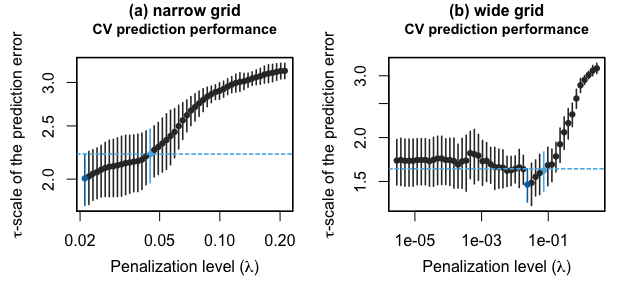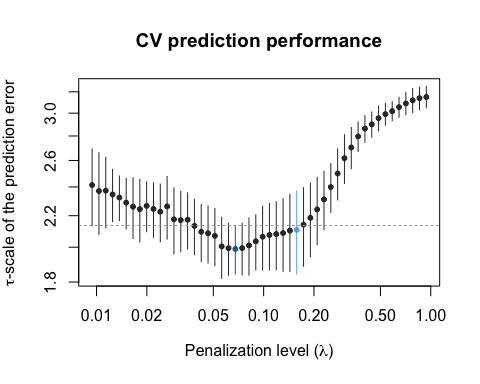# Controlling the grid of penalization levels

#### 2021-04-14

The functions to compute (adaptive) PENSE and PENSEM estimates automatically select a grid of penalization levels. In many cases, this grid is well focused on the interesting range of penalization levels, but sometimes it needs to be adjusted. This guide shows how to adjust the grid of penalization levels for the the function adapense_cv(), i.e., for computing adaptive PENSE estimates. The steps, however, apply to all functions for computing robust regularized estimates in the pense package (using or not using cross-validation).

For this guide we first need to load the pense package:

library(pense)

For demonstration purposes, let’s simulate data with 50 observations and 40 available predictors. The error distribution is a heavy-tailed t-distribution and only the first 3 predictors are truly relevant for predicting the response y:

set.seed(1234)
x <- matrix(rweibull(50 * 40, 2, 3), ncol = 40)
y <- 1 * x[, 1] - 1.1 * x[, 2] + 1.2 * x[, 3] + rt(nrow(x), df = 2)

To make the demonstration more realistic, let’s add some contamination to the response value of the first 3 observations and to some predictors:

y[1:3] <- 5 * apply(x[1:3, ], 1, max)
x[3:6, 4:6] <- 1.5 * max(x) + abs(rcauchy(4 * 3))

## Adjusting the grid of penalization levels

By default, adapense_cv() creates a grid of 50 penalization levels. The grid spans from the “largest” penalization level (i.e., the penalization level where the solution is the intercept-only model) to a multiple of 10-3 of this largest penalization level.

The number of grid points is controlled with argument nlambda=. The more grid points, the more models are explored, but computation time increases accordingly. For most applications “meaningful” change is only observed between models differing in at least one predictor.

Particularly in the lower end of the grid, the estimated models often don’t differ in the number of predictors and exhibit only marginal differences in the coefficient estimates. This may waste computational resources on exploring an area of little interest. You can adjust the lower endpoint of the automatic grid by changing the multiplier with argument lambda_min_ratio=. The ratio must be less than 1, and the larger (i.e., closer to 1), the narrower the grid. If you need to re-focus the grid on larger values of the penalization level, you can increase the ratio to 10-1:

set.seed(1234)
fit_grid_narrow <- adapense_cv(x, y, alpha = 0.75, lambda_min_ratio = 1e-1, cv_k = 5, cv_repl = 10)

If the model at the lowest penalization level is still fairly sparse and the best model seems to be in this range, you may need to decrease the ratio, e.g., to 10-4 or even to 10-6:

set.seed(1234)
fit_grid_wide <- adapense_cv(x, y, alpha = 0.75, lambda_min_ratio = 1e-6, cv_k = 5, cv_repl = 10)Prediction performance of models estimated on different grids of the penalization level: (a) narrow grid with lambda_min_ratio=1e-1, (b) wide grid with lambda_min_ratio=1e-6.

From these plots we can see that the grid in the left plot is too narrow, as a smaller penalization level than 0.02 would likely lead to a better predictive model. On the right, however, the grid is too wide. Penalization levels less than 10-3 lead to very similar estimates with similar prediction accuracy, while the range with meaningful change seems to be covered too coarsely.

From this, we can compute adaptive PENSE estimates on a better focused grid with:

set.seed(1234)
fit_grid_focused <- adapense_cv(x, y, alpha = 0.75, lambda_min_ratio = 1e-2, cv_k = 5, cv_repl = 10)

Indeed, the plot shows that the range of interest (around the minimum) is covered by several penalization levels:Prediction performance of models estimated over a well-focused grid of penalization levels.

### Manually supplying a grid of penalization levels

The function adapense_cv() and friends also allows you to specify your own grid of penalization levels via the lambda= argument.

Note that for adapense_cv() the argument lambda= applies to both the preliminary PENSE estimate and the adaptive PENSE estimate. In most cases, however, this is not what you want. A sensible grid of penalization levels for the preliminary estimate is in general quite different from a good grid of penalization levels for the adaptive estimate.

To compute adaptive PENSE estimates with separately specified manual grids for the preliminary and the adaptive PENSE estimates, you need to compute them manually via

fit_preliminary <- pense_cv(x, y, alpha = 0, cv_k = 5, cv_repl = 10, lambda = c(1e-5, 1e-4, 1e-3, 1e-2, 1e-1, 1))
exponent <- 1
fit_adaptive <- pense_cv(x, y, alpha = 0.75, cv_k = 5, cv_repl = 10, lambda = c(5e-5, 5e-4, 5e-3, 5e-2, 5e-1, 5))
The function pensem_cv() allows finer control over the grids. It supports the argument lambda=, which would use the same grid of penalization levels for the S- and M-estimates. But pensem_cv() additionally supports arguments lambda_s= and lambda_m= to manually specify separate grids for the S- and the M-estimates.